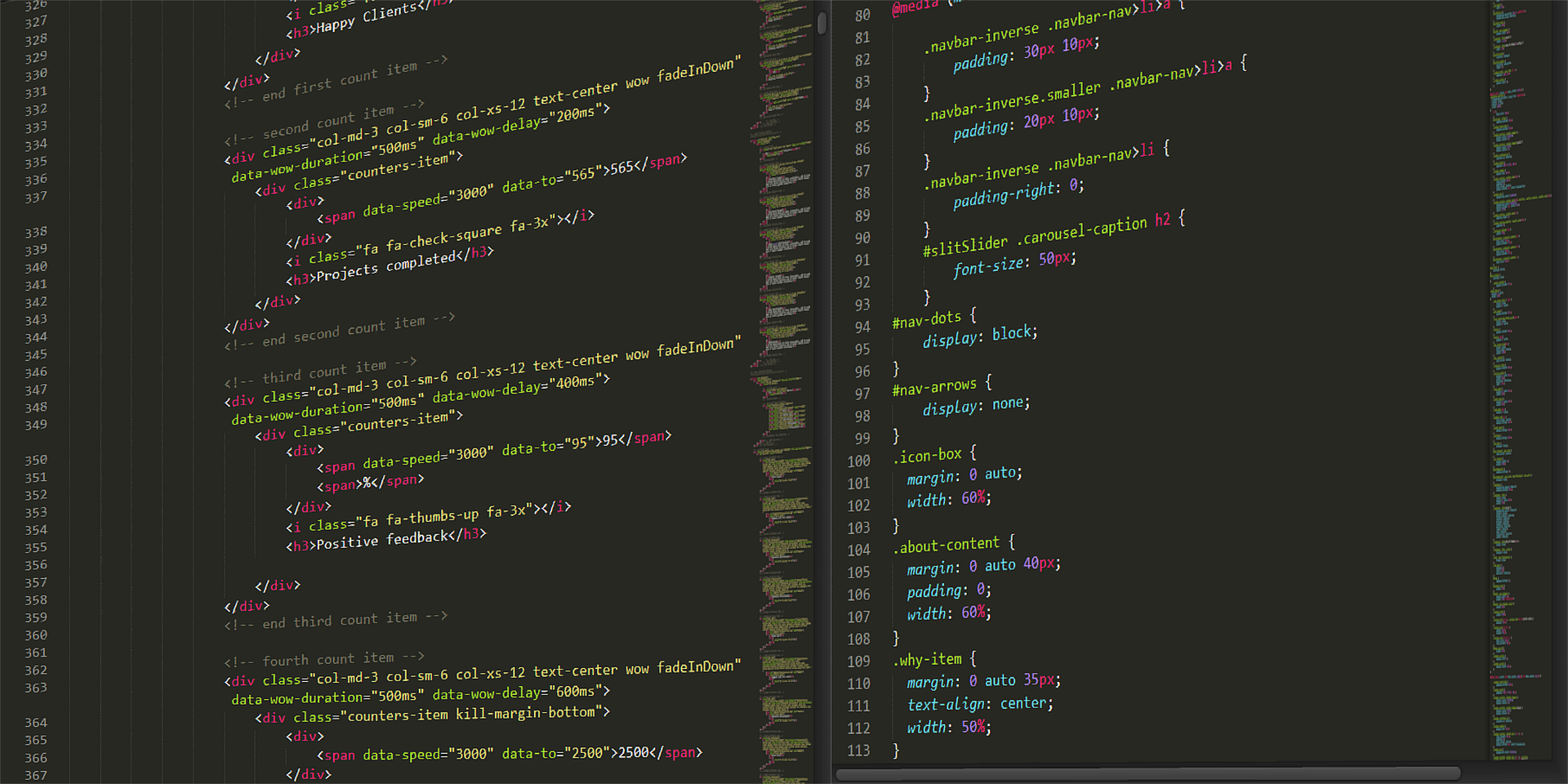Posted on

Data Combination; C has a rich set of operators for manipulating and combining values. The principal operators are 1. Arithmetic operators. C provides: SEC. 1.4 THE C DATA MODEL 19 a) The customary binary arithmetic operators +, −, ∗, / on integers and floating-point numbers. Integer division truncates (4/3 yields 1). b) There are the unary + and − operators. c) The modulus operator I % j produces the remainder when i is divided by j. d) The increment and decrement operators, ++ and –, applied to a single integer variable add or subtract 1 from that variable, respectively. These operators can appear before or after their operand, depending on whether we wish the value of the expression to be computed before or after the change in the variable’s value. 2. Logical operators. C does not have a Boolean type; it uses zero to represent the logical value false, and nonzero to represent true.5 C uses: a) && to represent AND.For example, the expression x && y returns 1 if both operands are nonzero, 0 otherwise. However, y is not evaluated if x has the value 0. b) || represents OR. The expression x || y returns 1 if either x or y is nonzero, and returns 0 otherwise. However, y is not evaluated if x is nonzero. c) The unary negation operator !x returns 0 if x is nonzero and returns 1 if x = 0. d) The conditional operator is a ternary (3-argument) operator represented by a question mark and a colon. The expression x?y:z returns the value of y if x is true (i.e., it is nonzero) and returns the value of z if x is false (i.e., 0). 3. Comparison operators. The result of applying one of the six relational comparison operators (==, !=, <, >, <=, and >=) to integers or floating point numbers is 0 if the relation is false and 1 otherwise. 4. Bitwise manipulation operators.

C provides several useful bitwise logical operators, which treat integers as if they were bits strings equal to their binary representations. These include & for bitwise AND, | for bitwise inclusive-or, ^ for bitwise exclusive-or, << for left shift, >> for right shift, and a tilde for left shift. 5. Assignment operators. C uses = as the assignment operator. In addition, C allows expressions such as x = x + y; to be written in a shortened form x += y; Similar forms apply to the other binary arithmetic operators.

𐌢